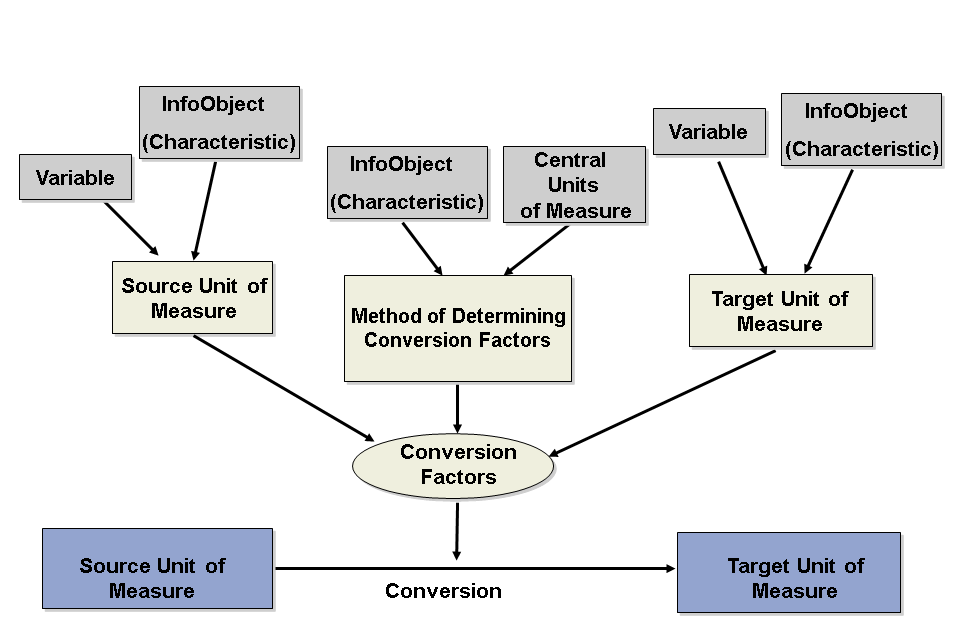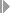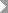Show TOC

# Quantity Conversion TypesDefinition

A quantity conversion type is a combination of different parameters that establish how the conversion is performed.

Structure

The parameters that determine the conversion factors are the source and target unit of measure and the option you choose for determining the conversion factors.The decisive factor in defining a conversion type is the way in which you want conversion factors to be determined. Entering source and target quantities is optional.

Conversion Factors

The following options are available:

• Using reference InfoObject

The system tries to determine the conversion factors from the reference InfoObject you have chosen or from the associated quantity DataStore object.

If you want to convert 1000 grams into kilograms but the conversion factors are not defined in the quantity DataStore object, the system cannot perform the conversion, even though this is a very simple conversion.

• Using central units of measure (T006)

Conversion is performed using the function module UNIT_CONVERSION_SIMPLE using unit of measure table T006. Conversion can only take place if the source unit of measure and target unit of measure belong to the same dimension (for example, meters to kilometers, kilograms to grams, and so on).

• Using reference InfoObject if available, central units of measure (T006) if not

The system tries to determine the conversion factors using the quantity DataStore object you have defined. If the system finds conversion factors, it uses these to perform the calculation. If the system cannot determine conversion factors from the quantity DataStore object it tries again using table T006 for units of measure.

• Using central units of measure (T006) if available, reference InfoObject if not

The system tries to find the conversion factors in table T006 for units of measure. If the system finds conversion factors it uses these to perform the conversion. If the system cannot determine conversion factors from the central units of measure it tries to find conversion factors that match the attributes of the data record by looking in the quantity DataStore object.

• Using SI units (/ISQC/C_UT)

The conversion is performed using measurements stored in table /ISQC/C_UT. These are the measurements of the international unit system (SI, abbreviation of: Le Système international d'unités). This conversion is also used in ERP using class /ISQC/CL_QTY_CONVERSION with method UNIT_CONVERSION.

• Using reference InfoObject if available, SI units (/ISQC/C_UT) if not

The system tries to determine the conversion factors using the quantity DataStore object you have defined. If the system finds conversion factors, it uses these to perform the calculation. If the system cannot determine conversion factors from the quantity DataStore object it tries again using table /ISQC/C_UT.

• Using SI units (/ISQC/C_UT) if available, reference InfoObject if not

The system tries to find the conversion factors in table /ISQC/C_UT for SI units. If the system finds conversion factors it uses these to perform the conversion. If the system cannot determine conversion factors from the central units of measure it tries to find conversion factors that match the attributes of the data record by looking in the quantity DataStore object.

The settings that you can make in this regard affect performance and the decision must be strictly based on the data set.

If you only want to perform conversions within the same dimension, option 2 is most suitable.

If you are performing InfoObject-specific conversions (for example, material-specific conversions) between units that do not belong to the same dimension, option 1 is most suitable.

In both cases, the system only accesses one database table. That table contains the conversion factors.

With option 3 and option 4, the system tries to determine conversion factors at each stage. If conversion factors are not found in the central table (T006), the system searches again in the quantity DataStore object, or in reverse.

The option you choose should depend on how you want to spread the conversion. If the source unit of measure and target unit of measure belong to the same dimension for 80% of the data records that you want to convert, first try to determine factors using the central units of measure (option 4), and accept that the system will have to search in the second table also for the remaining 20%.

The Conversion Factor from InfoObject option (as with Exchange Rate from InfoObject in currency translation types) is only available when you load data. The key figure you enter here has to exist in the InfoProvider and the attribute this key figure has in the data record is taken as the conversion factor.

Source Unit of Measure

The source unit of measure is the unit of measure that you want to convert. The source unit of measure is determined dynamically from the data record or from a specified InfoObject (characteristic). In addition, you can specify a fixed source unit of measure or determine the source unit of measure using a variable.

When converting quantities in the Business Explorer, the source unit of measure is always determined from the data record.

During the data load process the source unit of measure can be determined either from the data record or using a specified characteristic that bears master data.

You can use a fixed source unit of measure in planning functions. Data records are converted that have the same unit key as the source unit of measure.

The values in input help correspond to the values in table T006 (units of measure).

You access maintenance for the unit of measure inSAP Customizing Implementation GuideSAP NetWeaverGeneral SettingsCheck Units of Measure.

In reporting, you can use a source unit of measure from a variable. The variables that have been defined for InfoObject 0UNIT are used.

Target Unit of Measure

You have the following options for determining the target unit of measure:

• You can enter a fixed target unit of measure in the quantity conversion type (for example, 'UNIT').

• You can specify an InfoObject in the quantity conversion type that is used to determine the target unit of measure during the conversion. This is not the same as defining currency attributes where you determine a currency attribute on the Business Explorer tab page in characteristic maintenance. With quantity conversion types you determine the InfoObject in the quantity conversion type itself. Under InfoObject for Determining Unit of Measure, all InfoObjects are listed that have at least one attribute of type Unit. You have to select one of these attributes as the corresponding quantity attribute.

• Alternatively, you can determine that the target unit of measure be determined during the conversion. In the Query Designer under the properties for the relevant key figure, you specify either a fixed target unit of measure or a variable to determine the target unit of measure.

• Target quantity using InfoSet

This setting covers the same functionality as InfoObject for Determining Target Quantity. If the InfoObject that you want to use to determine the target quantity is unique in the InfoSet (it only occurs once in the whole InfoSet), you can enter the InfoObject under InfoObject for Determining Target Quantity.

You only have to enter the InfoObject in Target Quantity Using InfoSet if you want to determine the target quantity using an InfoObject but that occurs more than once in the InfoSet.

Example

The InfoSet contains InfoProviders A and B and both A and B contain InfoObject X with a quantity attribute. In this case you have to specify exactly whether you want to use X from A or X from B to determine the target quantity. Field aliases are used in an InfoSet to ensure uniqueness.

All the active InfoSets in the system can be displayed using input help. As long as you have selected an InfoSet, you can select an InfoObject. All the InfoObjects with quantity attributes contained in the InfoSet can be displayed using input help.